# NCERT Solutions - Motion, Science, Class 9 Notes | Study Class 9 Science by VP Classes - Class 9

## Class 9: NCERT Solutions - Motion, Science, Class 9 Notes | Study Class 9 Science by VP Classes - Class 9

The document NCERT Solutions - Motion, Science, Class 9 Notes | Study Class 9 Science by VP Classes - Class 9 is a part of the Class 9 Course Class 9 Science by VP Classes.
All you need of Class 9 at this link: Class 9

Q1. An object has moved through a distance. Can it have zero displacement? If yes, support your answer with an example.
Ans.
Yes, the object inspite of moving through a distance can have zero displacement.
Example, if an object travels from point ‘A’ and reaches to the same point ‘A’, then its displacement is ‘0’.

Q2. A farmer moves along the boundary of a square field of side 10 m in 40 s. What will be the magnitude of displacement of the farmer at the end of 2 minutes 20 seconds from his initial position?
Ans.
Total distance = perimeter of square = 4 × 10 m = 40 m

Time taken = 40 s

∴ Total time taken by the farmer = 2 min 20 seconds = 140 seconds

∴ 140 ÷ 40 = 3.5

∴ The farmer completes 3 and a half round of the square. i.e., if he starts from A he reaches point ‘C’ after 2 minutes 20 seconds.

∴ Displacement is AC.

To find AC, assume ∆ADC (right angle triangle)

AC2 = (10)2 + (10)2

AC2 = 100 +100

AC= 200

∴ AC = 10√2 m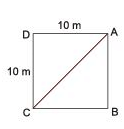Q3. Which of the following is true for displacement?
(a) It cannot be zero.
(b) Its magnitude is greater than the distance travelled by the object.
Ans.
(a) False
(b) False

Q4. Distinguish between speed and velocity.
Ans.

 Speed Velocity 1.The distance travelled by a moving body per unit time is called its speed. 1.The distance travelled by moving body in a particular direction per unit time is velocity. 2. It is scalar quantity. 2. It is a vector quantity.

Q5. Under what condition(s) is the magnitude of average velocity of an object equal to its average speed?
Ans.
The magnitude of average velocity of an object is equal to its average speed if the object moves in a straight line in a particular direction.

Q6. What does the odometer of an automobile measure?
Ans.
The odometer of an automobile measure the distance travelled by a vehicle.

Q7. What does the path of an object look like when it is in uniform motion?
Ans.
It is a straight line.

Q8. During an experiment, a signal from a spaceship reached the ground station in five minutes. What was the distance of the spaceship from the ground station? The signal travels at the speed of light, that is 3 × 108 ms–1.
Ans.
Time taken = 5 minutes = 5 × 60 sec = 300 sec Speed of signal u = 3 × 108 m/s Distance = ?

Speed = distance/ time         ∴ distance = speed x time

∴ Distance = 3 x 108 x 300

9 x 1010 m

Q9. When will you say a body is in (i) uniform acceleration? (ii) non-uniform acceleration?
Ans.
(i) Uniform acceleration: If the velocity of a body changes by equal amounts in equal interval of time, howsoever small or large these intervals may be, the acceleration is said to be uniform. Acceleration due to gravity is an example of uniform acceleration.
(ii) Non-uniform acceleration: If velocity changes by unequal amount in equal interval of time or if the direction of motion changes then the acceleration is said to be non-uniform. Acceleration of a train running from one station to another is a non-uniform acceleration.

Q10. A bus decreases its speed from 80 km h–1 to 60 km h–1 in 5 s. Find the acceleration of the bus.
Ans.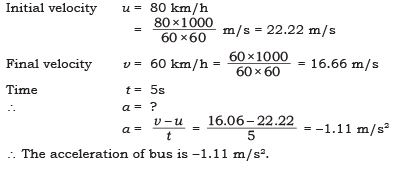Q11. A train starting from a railway station and moving with uniform acceleration attains a speed 40 km h–1 in 10 minutes. Find its acceleration.
Ans.
u = 0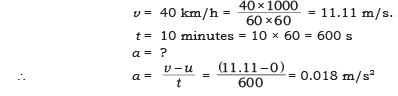Q12. What is the nature of the distance-time graphs for uniform and non-uniform motion of an object?
Ans.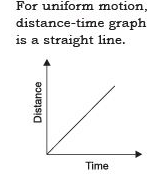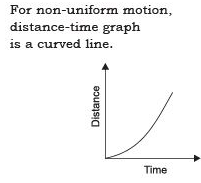Q13. What can you say about the motion of an object whose distance-time graph is a straight line parallel to the time axis?
Ans.
The body is at rest.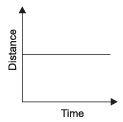Q14. What can you say about the motion of an object if its speed-time graph is a straight line parallel to the time axis?
Ans.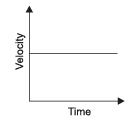The body is moving with a uniform speed and zero acceleration.

Q15. What is the quantity which is measured by the area occupied below the velocity-time graph?
Ans.
Distance travelled.

Q16. A bus starting from rest moves with a uniform acceleration of 0.1 ms–2 for 2 minutes. Find
(a) the speed acquired,
(b) the distance travelled.
Ans.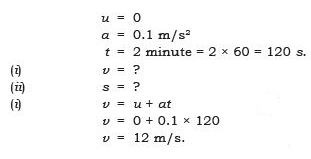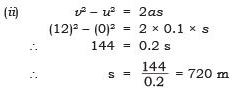The speed acquired is 12 m/s and the distance travelled is 720 m.
The speed acquired is 12 m/s and the distance travelled is 720 m.

Q17. A train is travelling at a speed of 90 km h–1. Brakes are applied so as to produce a uniform acceleration of −0.5 ms–2. Find how far the train will go before it is brought to rest.
Ans.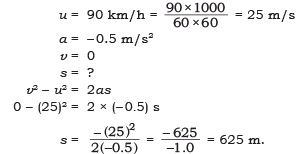Q18. A trolley, while going down an inclined plane, has an acceleration of 2 cm s–2. What will be its velocity 3 s after the start?
Ans.

a = 2 cm/s
t = 3 s v = ?
u = 0
v = u + at
= 0 + 2 × 3
v = 6 cm/s.

The velocity of a trolley 3 s after the start will be 6 cm/s.

Q19. A racing car has a uniform acceleration of 4 ms–2. What distance will it cover in 10 s after start?
Ans.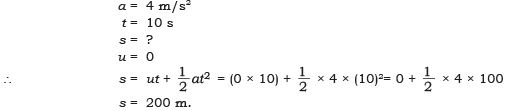The distance covered in 10 s by the car is 200 m.

Q20. A stone is thrown in a vertically upward direction with a velocity of 5 ms–1. If the acceleration of the stone during its motion is 10 ms–2 in the downward direction, what will be the height attained by the stone and how much time will it take to reach there?
Ans.

u = 5 m/s
v= 0
a= -10m/s2

s= ?
t = ?
v= u+at
O = 5+(-10)t
-5 = -10t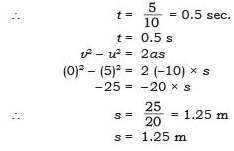The height attained by the stone is 1.25 m and time required to reach there is 0.5 s.

NCERT Textual Questions Solved

Q1. An athlete completes one round of a circular track of diameter 200 m in 40 s. What will be the distance covered and the displacement at the end of 2 minutes 20 s?
Ans.
Diameter = 200 m, r = (d/2)= 100 m

Time for one round = 40 s

Distance travelled in 2 minutes and 20 s

= (2 × 60) + 20 = 140 s

∴    (140/40) = 3.5 rounds

Distance travelled = Circumference of the circle × 3.5

= 2 πr × 3.5 = 2 × (22/7)× 100 × 3.5 = 2200 m

Displacement after 3.5 revolution = diameter of the track = 200 m.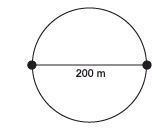Q2. Joseph jogs from one end A to the other end B of a straight 300 m road in 2 minutes 30 seconds and then turns around and jogs 100 m back to point C in another 1 minute. What are Joseph’s average speeds and velocities in jogging
(a) from A to B and
(b) from A to C?
Ans
. (a) From A to B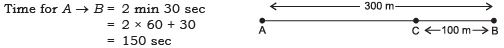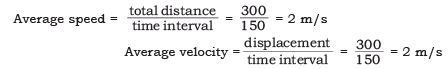(b) From A to C
Total distance = A to B + B to C = (300 + 100) = 400 m
Time taken = 150 + 60 = 210 sec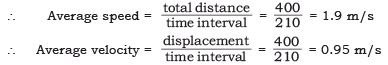Q3. Abdul, while driving to school, computes the average speed for his trip to be 20 km h–1. On his return trip along the same route, there is less traffic and the average speed is 30 km h–1. What is the average speed for Abdul’s trip?
Ans.

Average speed = (time/ total distance)

∴ Let the distance from Abdul’s home to school = x km
∴ While driving to school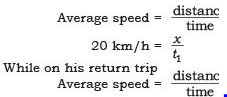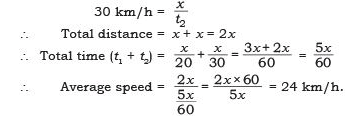Q4. A motorboat starting from rest on a lake accelerates in a straight line at a constant rate of 3.0 ms–2 for 8.0 s. How far does the boat travel during this time?
Ans.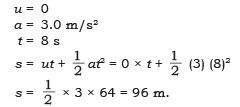∴ Boat travelled a distance of 96 m.

Q5. A driver of a car travelling at 52 km h–1 applies the brakes and accelerates uniformly in the opposite direction. The car stops in 5 s. Another driver going at 3 km h–1 in another car applies his brakes slowly and stops in 10 s. On the same graph paper plot the speed versus time graphs for the two cars. Which of the two cars travelled farther after the brakes were applied?
Ans
. Initial speed of first driver =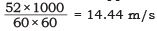Initial speed of second driver =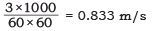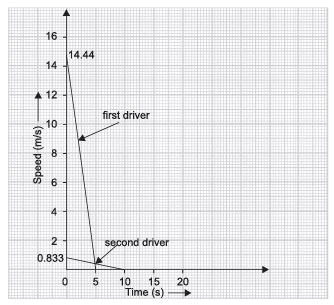Distance covered by first driver = (1/2)× 14.44 × 5 = 36.1 m

Distance covered by second driver = (1/2)× 0.833 × 10 = 4.165 m

Hence, distance travelled by first car is more.

Q6. Figure given below shows the distance-time graph of three objects A, B and C, study the graph and answer the following questions.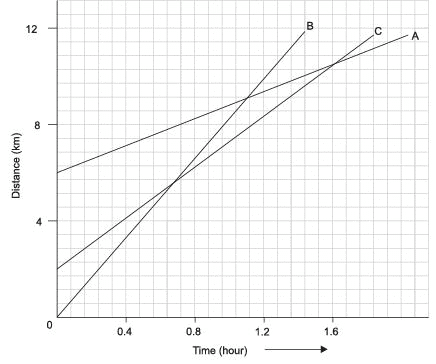(a) Which of the three is travelling the fastest?
(b) Are all three ever at the same point on the road?
(c) How far has C travelled when B passes A?
(d) How far has B travelled by the time it passes C?
Ans.
(a) B is travelling fastest.
(b) No, three lines do not meet at any point.
(c) When B passes A, C is at a distance approximately 8 km from origin.
(d) By the time B passes C, it has travelled 5.5 km.

Q7. A ball is gently dropped from a height of 20 m. If its velocity increases uniformly at the rate of 10 ms–2, with what velocity will it strike the ground? After what time will it strike the ground?
Ans.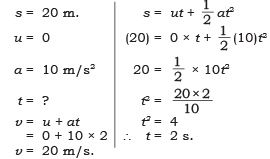The ball will strike the ground after 2 s with the velocity of 20 m/s.

Q8. The speed-time graph for a car is shown in the figure given below.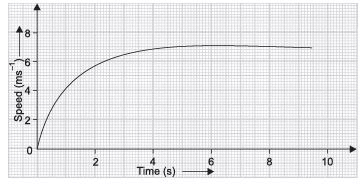(a) Find how far does the car travel in the first 4 seconds. Shade the area on the graph that represents the distance travelled by the car during the period.
(b) Which part of the graph represents uniform motion of the car?
Ans.
(a) The distance travelled by car in the first 4 seconds is approximately 12 m.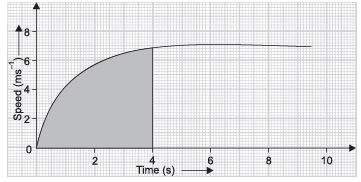(b) The uniform motion of the car is shown after 5.5 s.

Q9. State which of the following situations are possible and give an example for each of these:
(a) an object with a constant acceleration but with zero velocity
(b) an object moving with an acceleration but with uniform speed
(c) an object moving in a certain direction with an acceleration in the perpendicular direction.
Ans.
(a) Free fall due to gravity
(b) Not possible
(c) Object moving in a circular path

Q10. An artificial satellite is moving in a circular orbit of radius 42250 km. Calculate its speed if it takes 24 hours to revolve around the earth.
Ans.
Radius of the orbit = 42250 km = 42250 × 1000 m

Time taken for one revolution = 24 hours = 24 × 60 × 60 sec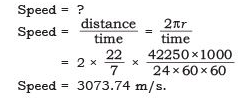The document NCERT Solutions - Motion, Science, Class 9 Notes | Study Class 9 Science by VP Classes - Class 9 is a part of the Class 9 Course Class 9 Science by VP Classes.
All you need of Class 9 at this link: Class 9Use Code STAYHOME200 and get INR 200 additional OFF

## Class 9 Science by VP Classes

75 docs|14 tests

Track your progress, build streaks, highlight & save important lessons and more!

,

,

,

,

,

,

,

,

,

,

,

,

,

,

,

,

,

,

,

,

,

,

,

,

,

,

,

;